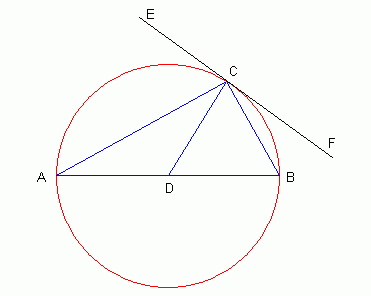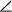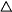Question from Namrata: AB is a diameter and AC IS A CHORD OF A CIRCLE SUCH THAT angle=30. the tangent at C intersect AB produced in a pointD. Prove that BC=BD. Hello Namrata, I tried drawing a diagram based on your description and this is the best I could do:As you can see, I have drawn the tangent line to the circle at location C (I've called it the line EF). But you can see from the diagram that the tangent line EF doesn't intersect the diameter AB. I think what you meant to say was the perpendicular to the tangent at C intersects AB. That's what I've drawn, because the rest makes sense then. The first step is in recognizing that a perpendicular to any tangent of a circle must pass through the center point of the circle. As well, any diameter of a circle must pass through the center point of the circle. That means that point D must be the center of the circle. The second step is remembering the property that the measure ofCAB must be half ofCDB. This is because they subtend the same arc on the circle, and any time that happens, the angle on the circumference is half the angle from the center. That means thatCDB = 2(30o ) = 60o . The third step is realizing that any line segment from the center to the circumference is a radius, so this means CD = BD. The last step is looking at triangleCDB and recognizing that it is an isoceles triangle. Since you know the included angleCDB is 60o, this gives you enough information to determine the relationships between any side lengths, such as BC and BD. Hope this helps, Stephen La Rocque.